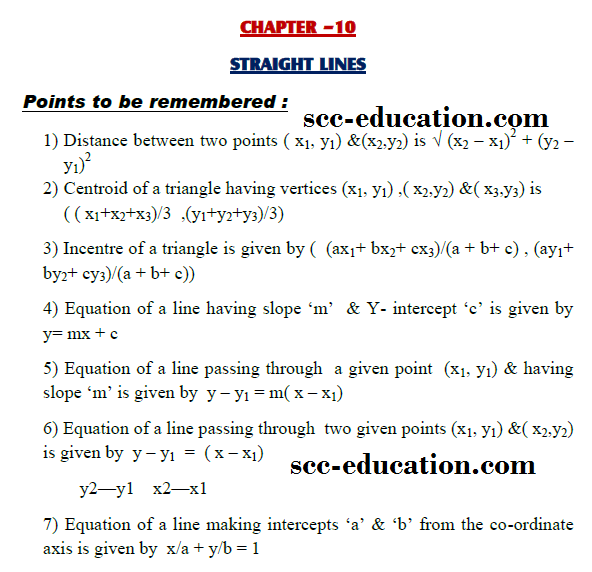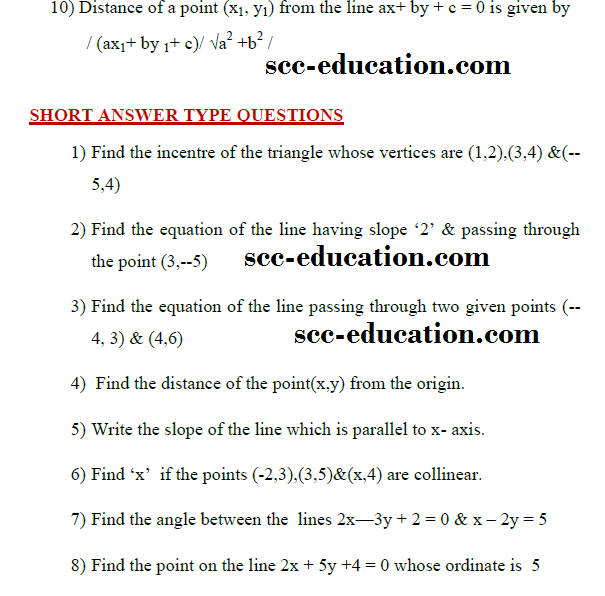# SCC Education

## Straight line,intercept form,perpendicular form,in centre,angle between line

Straight line
Important questionsSeries-formulas

The-binomial-theorem

solved-problems-on-limits-and-continuity

geometric-progressions

Basic-point-formulas-distance-midpoint

Two-point-form-normal-form parametric

limit-questions

set-relation-function-part1
permutation-combination

straight-lines

Three-diamensional-geometry

Trignometry

hyperbola